###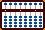SUBTRACTION EXAMPLES

The Bead Unbaffled - An Abacus Manual

**All of the following examples will require using complementary numbers. In order to fully understand the technique, it's best to work these examples using your abacus.**

Example: 102 - 78 = 24```Step 1 A B C . 1 0 2 ``` ` ```` Step 2 A B C . 1 0 2 -1 Step 2 0 0 2 +3   Step 2a 0 3 2```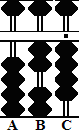```Step 3 A B C . 0 3 2 -1 Step 3 0 2 2   +2 Step 3a 0 2 4```

Step 1: Set 102 on rods ABC.

Step 2: Subtract 7 from tens rod B. Use the complement. Begin by subtracting 1 from hundreds rod A, then....
Step 2a: Add the complementary 3 to rod B leaving 32 on rods BC.

Step 3: Subtract 8 from units rod C. Use the complement again. Begin by subtracting 1 from hundreds rod A, then....
Step 3a and the answer: Add the complementary 2 to rod C leaving the answer 24 on rods BC.

Example: 146 - 57 = 89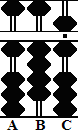```Step 1 A B C . 1 4 6 ``` ` `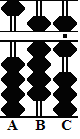``` Step 2 A B C . 1 4 6 -1 Step 2 0 4 6 +5   Step 2a 0 9 6```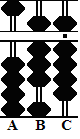```Step 3 A B C . 0 9 6 -1 Step 3 0 8 6   +3 Step 3a 0 8 9```

Step 1: Set 146 on rods ABC.

Step 2: Subtract 5 from tens rod B. Use the complement. Begin by subtracting 1 from hundreds rod A, then....
Step 2a: Add the complementary 5 to rod B, leaving 96 on rods ABC.

Step 3: Subtract 7 from units rod C. Use the complement again. Begin by subtracting 1 from hundreds rod A, then....
Step 3a and the answer: Add the complement 3 to rod C leaving the answer 89 on rods ABC.

Note the decimal numbers in the following example. Rod B is the designated unit rod.

Example: 22.3 - 2.8 = 19.5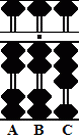```Step 1 A B C .   2 2 3 ``` ` `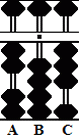```Step 2 A B C .   2 2 3 -2   Step 2 2 0 3``` ` `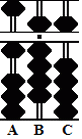``` Step 3 A B C .   2 0 3 -1 Step 3 1 0 3 +9 Step 3a 1 9 3  +2 Step 3b 1 9 5```

Step 1: Designate rod B as the unit rod. Set 22.3 on rods ABC.

Step 2: Subtract 2 from units rod B leaving 20.3 on rods ABC.

Step 3: Subtract 8 from tenths rod C. Use the complement. Begin by subtracting 1 from units rod on B...There's a problem. Rod B is empty. There's nothing there to subtract so we have to go all the way over to rod A for help.
Step 3a: Subtract 1 from tens rod A. Add 9 to rod B, *then*
Step 3b and the answer: Add the complementary 2 to rod C leaving 19.5 on rods ABC.

**Something like this last example may seem a stretch at first.**
But with a little practice, solving problems of this sort soon becomes second nature.

Print Page (.pdf format,100kb)